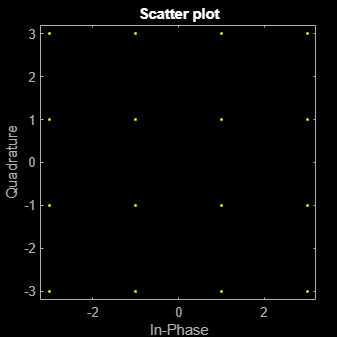# comm.RectangularQAMTCMModulator

Convolutionally encode binary data and map using rectangular QAM signal constellation

## Description

The `RectangularQAMTCMModulator` object implements trellis-coded modulation (TCM) by convolutionally encoding the binary input signal and mapping the result to a rectangular QAM signal constellation.

To convolutionally encode binary data and map the result using a rectangular QAM constellation:

1. Define and set up your rectangular QAM TCM modulator object. See Construction.

2. Call `step` to modulate the signal according to the properties of `comm.RectangularQAMTCMModulator`. The behavior of `step` is specific to each object in the toolbox.

Note

Starting in R2016b, instead of using the `step` method to perform the operation defined by the System object™, you can call the object with arguments, as if it were a function. For example, ```y = step(obj,x)``` and `y = obj(x)` perform equivalent operations.

## Construction

`H = comm.RectangularQAMTCMModulator` creates a trellis-coded, rectangular, quadrature amplitude (QAM TCM) System object, `H`. This object convolutionally encodes a binary input signal and maps the result to a rectangular QAM constellation.

`H = comm.RectangularQAMTCMModulator(Name,Value)` creates a rectangular QAM TCM modulator object, `H`, with each specified property set to the specified value. You can specify additional name-value pair arguments in any order as (`Name1`,`Value1`,...,`NameN`,`ValueN`).

`H = comm.RectangularQAMTCMModulator(TRELLIS,Name,Value)` creates a rectangular QAM TCM modulator object, `H`. This object has the `TrellisStructure` property set to `TRELLIS` and the other specified properties set to the specified values.

## Properties

 `TrellisStructure` Trellis structure of convolutional code Specify trellis as a MATLAB structure that contains the trellis description of the convolutional code. Use the `istrellis` function to check whether a structure is a valid trellis. The default is the result of `poly2trellis`(```[3 1 1]```, `[ 5 2 0 0; 0 0 1 0; 0 0 0 1]`). `TerminationMethod` Termination method of encoded frame Specify the termination method as `Continuous` | `Truncated` | `Terminated`. The default is `Continuous`. When you set this property to `Continuous`, the object retains the encoder states at the end of each input vector for use with the next input vector. When you set this property to `Truncated`, the object treats each input vector independently. The encoder is reset to the all-zeros state at the start of each input vector. When you set this property to `Terminated`, the object treats each input vector independently. For each input vector, the object uses extra bits to set the encoder to the all-zeros state at the end of the vector. For a rate K/N code, the `step` method outputs the vector with a length given by $y=N×\left(L+S\right)}{K}$, where S = constraintLength–1 (or, in the case of multiple constraint lengths, S = sum(constraintLength(i)–1)). L is the length of the input to the `step` method. `ResetInputPort` Enable modulator reset input Set this property to true to enable an additional input to the step method. The default is `false`. When you set the reset input to the step method to a nonzero value, the object resets the encoder to the all-zeros state. This property applies when you set the `TerminationMethod` property to `Continuous`. `ModulationOrder` Number of points in signal constellation Specify the number of points in the signal constellation used to map the convolutionally encoded data as a positive integer scalar value equal to `4`, `8`, `16`, `32`, or `64`. The default is `16`. The value of the `ModulationOrder` property must equal the number of possible output symbols from the convolutional encoder of the QAM TCM modulator. Thus, the value for the `ModulationOrder` property must equal 2N for a rate K/N convolutional code. `OutputDataType` Data type of output Specify the output data type as one of `double` | `single`. The default is `double`.

## Methods

 step Convolutionally encode binary data and map using rectangular QAM constellation
Common to All System Objects
`release`

Allow System object property value changes

`reset`

Reset internal states of System object

## Examples

collapse all

Modulate data using rectangular 16-QAM TCM modulation and display the scatter plot.

Generate random binary data. The length of the data vector must be an integer multiple of the number of input streams into the encoder, log2(8) = 3.

`data = randi([0 1],3000,1);`

Create a modulator System object™ and use its `step` function to modulate the data.

```hMod = comm.RectangularQAMTCMModulator; modData = step(hMod,data);```

Plot the modulated data.

`scatterplot(modData)`## Algorithms

This object implements the algorithm, inputs, and outputs described on the Rectangular QAM TCM Encoder block reference page. The object properties correspond to the block parameters.